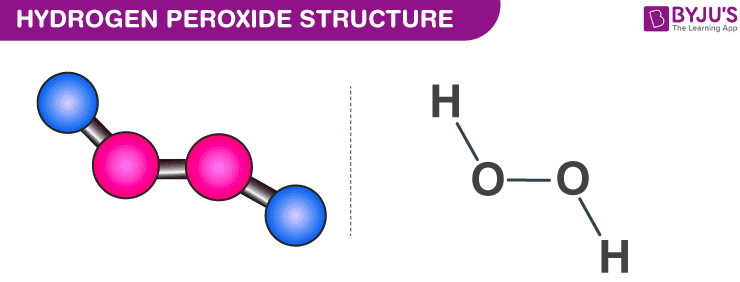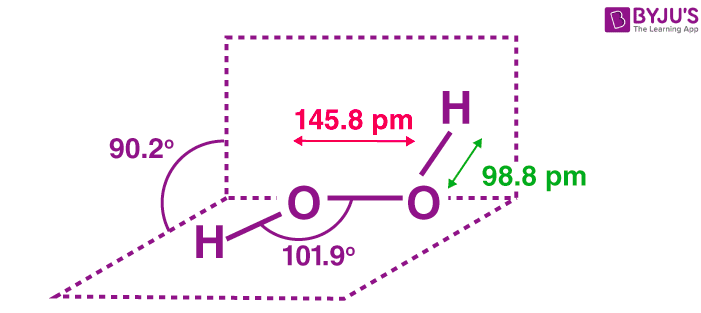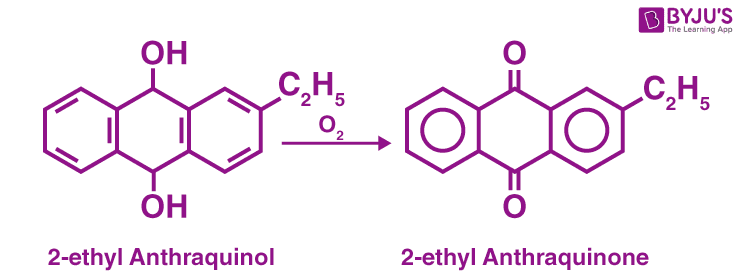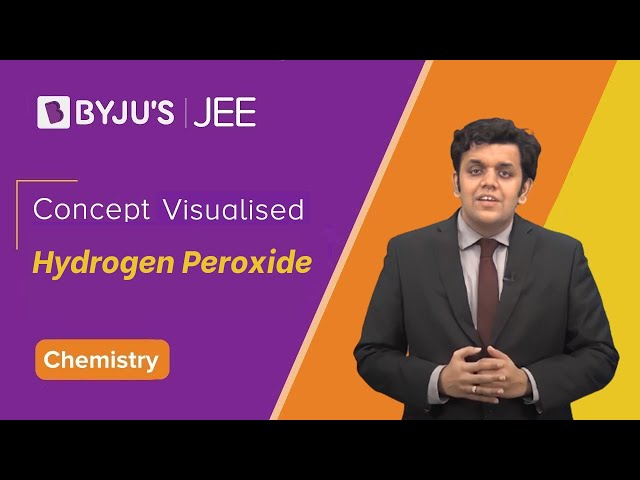Checkout JEE MAINS 2022 Question Paper Analysis : Checkout JEE MAINS 2022 Question Paper Analysis :

# Hydrogen Peroxide - Structure, Preparation, and Properties of H2O2

## What is Hydrogen Peroxide (H2O2)?

Hydrogen peroxide is the simplest kind of peroxide available (oxygen-oxygen single bond). It is a colourless liquid and is used in aqueous solution for safety reasons. It acts as a bleaching agent and is also used as a disinfectant. Concentrated hydrogen peroxide is a very reactive oxygen species and is used as a propellant in rocketry. The chemical formula for hydrogen peroxide is H2O2.

### Table of Content

It is often referred to as water with one more oxygen atom. It is acidic in nature and PH is about 4.5. It is 100 percent degradable compound.

##### Overview of H2O2 – Hydrogen Peroxide

 Hydrogen peroxide Chemical formula H2O2 Molecular Weight/ Molar Mass 4.0147 g/mol Density 1.05 g/cm3 Boiling Point 150.2 °C Melting Point -0.43 °C

## Structure of Hydrogen Peroxide

The structure of hydrogen peroxide is non-planar. H2O2 has an open book structure with O – O spins. The dihedral angle is 111°. The O-O bond length is 145.8 pm and the O-H bond length is 98.8 pm(which is equal to 9.88 × 10-13 m). The following diagram will clearly show what an open book structure means.Hydrogen Peroxide Structure

There are two planes in this structure and each plane has one O-H bond pair, the angle between both the planes is 90.2°.

## Preparation of Hydrogen Peroxide

Let us have a look at the various methods of preparation for hydrogen peroxide.

### Laboratory Methods of Preparation

When barium peroxide is acidified and the excess water is removed by the process of evaporation under reduced pressure, we obtain hydrogen peroxide. The following reaction will clarify this:

BaO2.8H2O(s) + H2SO4(aq) → BaSO4(s) + H2O2(aq) + 8H2O(l)

### Industrial Method of Preparation

Hydrogen peroxide is prepared by the electrolysis of 30% ice-cold H2SO4. When acidified sulfate solution is electrolyzed at high current density, peroxodisulphate is obtained. Peroxodisulphate is then hydrolyzed to get hydrogen peroxide.

2HSO4(aq) [Electrolysis] → HO3SOOSO3H(aq) [Hydrolysis] → 2HSO4(aq)+2H+(aq)+H2O2(aq)

#### Reaction Mechanism

• Electrolyte: 30% dilute H2SO4
• Cathode: Pb wire
• Anode: Pt rod

2H2SO4 → 2H+ 2HSO4

At Cathode: 2H+ 2e– → H2

At Anode:

2HSO→ H2S2O+ 2e– ⇒ Peroxodi Sulphuric Acid [Marshall’s acid]

H2S2O+ H2O → H2SO+ H2SO⇒ Peroxomono Sulphuric Acid [Caro’s acid]

H2SO+ H2O → H2SO+ H2O2

### Commercial Methods Of Preparation

#### Auto-Oxidation Method:Auto-Oxidation Method of Preparation of H2O2

## Properties of Hydrogen Peroxide

### Physical Properties:

• In the pure state, hydrogen peroxide is almost colourless (very pale blue) liquid.
• It melts at 272.4 K and has a boiling point of 423 K (extrapolated).
• It is miscible in water in all proportions and forms hydrates.

### Chemical Properties:

Hydrogen peroxide in both acidic and basic medium acts as an oxidizing as well as a reducing agent. The following reactions will give a clear picture:

#### Oxidation Reactions of H2O2

• Oxidizes black Pbs to white PbSO4. Reaction: Pbs + 4H2O2 → PbSO+ 4H2O
• Oxidises KI to Iodine. Reaction: 2KI + H2O→ 2KOH + I2
• Oxidizes nitrites to nitrates. Reaction: NaNO+ H2O2 → NaNO3 + H2O
• Oxidizes acidified Potassium ferrocyanide. Reaction: 2K4Fe(CN)6 + H2SO4 + H2O→ 2K3Fe(CN)6 + K2SO4 + 2H2O
• Oxidizes sulphites to sulphates. Reaction: Na2SO3 + H2O2 → Na2SO4 + H2O
• Oxidizes CrO-24 in an acid medium to chromium Peroxide CrO5Reaction: CrO-2+ 2H+ + 2H2O→ CrO5 + 3H2O

⇒ Check: Redox Reactions

#### Bleaching Reactions:

It bleaches by oxidation. H2O2 bleaches silk, wool, cotton, hair.

Reaction: H2O2 → H2O + [O] Nascent oxygen

Coloured substance + [O] → colourless

#### Reduction Reactions of Hydrogen Peroxide:

• It reduces Barium Peroxide to monoxide. Reaction: BaO2 + H2O2 → BaO + H2O + O2
• It reduces ozone to oxygen. Reaction: O+ H2O→ H2O + 2O2
• It reduces alkaline potassium ferricyanide. Reaction: 2K3Fe(CN)+ 2KOH + H2O→ 2K4Fe(CN)+ 2H2O + O2

### Why Hydrogen Peroxide is Stored in Plastic Containers?

Hydrogen peroxide decomposes when exposed to sunlight, this process is catalyzed by traces of alkali metals. Therefore, H2O2 is stored in wax-lined glass or plastic containers and kept in dark.

It should also be kept away from dust particles because dust can induce explosive decomposition of this compound.

### How to Determine the Concentration of Hydrogen Peroxide?

#### Volume Strength of H2O2

10 Vol H2O2 means 1 mL of H2O2 gives 10 mL of O2 at STP.

#### Weight Percentage [w/w%]

Let us consider 10 Vol H2O2

2H2O→ 2H2O + O2

→ 68g of H2O2 gives 22.4 L of O2 at STP.

→ 10 L of O2 is given by

Amount of hydrogen peroxide = 10 × 68/22.4 = 30.36 g

1000 mL of H2O2 → 30.36 g

100 mL → ?

wt% =

$$\begin{array}{l}\frac{1\cancel{00}\times 30.36}{10\cancel{00}} \end{array}$$

= 30.36%

100 Vol H2O2 or 3.036%(w/w) H2O2 is known as Perhydrol.

#### Molarity of H2O2

To calculate the molarity, Let us consider 10 Vol H2O2

M =

$$\begin{array}{l}\frac{wt\times 1000}{GMw\times Vin\,\,mL}\end{array}$$

Molarity of hydrogen peroxide = 30.36/34 = 0.89 M.

#### Normality of H2O2

Let us consider 10 Vol H2O2

N =

$$\begin{array}{l}\frac{wt\times 1000}{Gm.Eq.wt\times Vin\,mL}\end{array}$$

= 30.36/17 = 1.78N

Also Read: What is Hydrogen Peroxide Used For

#### Hydrogen Peroxide – Video Lesson## Frequently Asked Questions – FAQs

### What is hydrogen peroxide used for?

Hydrogen peroxide is used for stain removal, as a cleanser and as a disinfectant.

### State three physical properties of hydrogen peroxide.

The physical properties of hydrogen peroxide are:
It is a pale blue colour in its pure form.
The viscosity of H2O2 is slightly more than water.
The boiling point of hydrogen peroxide is 150.2°C.

### What is the oxidation of oxygen in hydrogen peroxide?

The oxidation state of oxygen in hydrogen peroxide is -1.

### Give an example of a reaction in which hydrogen peroxide is an oxidizing agent.

Hydrogen peroxide oxidizes sodium nitrate to sodium nitrite.
NaNO2 + H2O2 → NaNO3 + H2O

### What is the product of the reaction between ozone and hydrogen peroxide?

Ozone on reaction with hydrogen peroxide gives oxygen and water. Hydrogen peroxide acts as a reducing agent in this reaction.
O3 + H2O2 → H2O + 2O2

Test your knowledge on Hydrogen Peroxide Properties Preparation

#### 1 Comment

1. pushpendra jain

nice article.